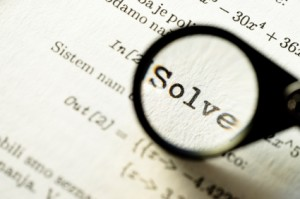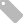# Doug Lemov's field notes

Reflections on teaching, literacy, coaching, and practice.

## 01.14.13Writing in Math ClassMy colleague Paul Powell has been working on a training for teachers on writing in math class—how to include more of it and how to use it to deepen understanding and rigor.  He’s done some great work in laying out and thinking about all the different types of writing that can happen in a math class and their comparative levels of rigor.  I took some of that material to make this list of the types of writing in math class.

What on this list have you used and how?  What’s missing from the list? How do you think about the relationships between the different types?

(Lightly Adapted) List of Types of Writing in Math Class (from Paul Powell)

Explanation of a Procedure

A triangle has angle A with a measure of 25°, Angle C with a measure of 60°.  What is the measure of Angle B?  Explain Your Work:

Correcting an Error

Tomfoolery simplified the expression and got an answer of 15.  Is Tom correct?  Explain why or why not.

Descriptive Observation

What does the attached graph tell you about the relationship to temperature and energy use?

Predictive Observation

How would the graph change if for one year the average temperature in every month was ten percent closer to the yearly average? How would the total amount of energy consumption change? How would the total amount of energy consumption change if for one year the average temperature in every month went down by ten percent?

Explanation of Concept

In the fraction ¼, what does the 4 represent?

Drafting Word Problems

Write a question that requires a multi-step equation with the variable x on both sides to solved.

Inferring a Rule

What can you conclude about the relationship between the size of the denominator and the value of a given fraction?

Comparing Two Correct Solutions

Which solution to the problem is more effective?  Explain why by citing specific parts of the solution.

Articulation of a Rule

Articulate the general rule for adding fractions by find the sum of fractions a/b and c/d where a, b, c, and d are all natural numbers., , ,